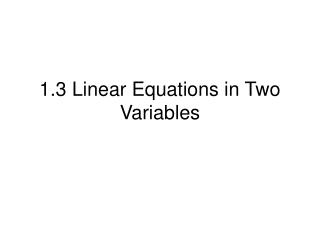# 1.3 Linear Equations in Two Variables - PowerPoint PPT PresentationDownload Presentation1.3 Linear Equations in Two Variables

1.3 Linear Equations in Two VariablesDownload Presentation## 1.3 Linear Equations in Two Variables

- - - - - - - - - - - - - - - - - - - - - - - - - - - E N D - - - - - - - - - - - - - - - - - - - - - - - - - - -
##### Presentation Transcript

1. 1.3 Linear Equations in Two Variables

2. Objective • Write linear equations in two variables. • Use slope to identify parallel and perpendicular lines. • Use slope and linear equations in two variables to model and solve real-life problems.

3. The Slope-intercept Form of the Equation of a Line • The graph of the equation • y = mx+b • is a line whose slope is m and whose y-intercept is (0, b)

4. A vertical line has an equation of the form x = a. • The equation of a vertical line cannot be written in the form y = mx + b because the slope of a vertical line is undefined.

5. Finding the Slope of a Line Passing Through Two Points • The slope m of the nonvertical line through

6. Example 1 Finding the Slope of a Line Through Two Points • Find the slope of the line passing through each pair of points. • a. (-5, -6) and (2,8) b. (3, 4) and (3, 1)

7. Writing Linear Equations in Two Variables • Point-Slope Form of the Equation of a Line • The equation of the line with slope m passing through the point

8. Example 2 Using the Point-Slope • Find the slope-intercept form of the equation of the line that has a slope of 2 and passes through the point (3, -7).

9. From the point-slope form we can determine the two-point form of the equation of a line.

10. Parallel and Perpendicular Lines • Two distinct nonvertical lines are parallel if and only if their slopes are equal. That is

11. Two nonvertical lines are perpendicular if and only if their slopes are negative reciprocals of each other. That is,

12. Example 3 Finding Parallel and Perpendicular Lines • Find the slope-intercept form of the equation of the line that passes through the point (-4, 1) and is (a) parallel to and (b) perpendicular to the line

13. Applications • In real-life problems, the slope of a line can be interpreted as either a ratio or rate of change. • If the x- and y-axis have the same unit of measure, then the slope has no units and is a ratio. • If the x-axis and y-axis have different units of measure, then the slope is a rate or rate of change.

14. Example 4 Using slope as a ratio • When driving down a mountainside, you notice warning signs indicating that the road has a grade of twelve percent. This means that the slope of the road is -12/100. Approximate the amount of horizontal change in your position if you note from elevation markers that you have descended 2000 feet vertically.

15. Example 5 • The following are the slopes of lines representing annual sales y in terms of time x in years. Use the slopes to interpret any change in annual sales for a 1-year increase in time • The line has a slope of m = 120. • The line has a slope of m = 0. c. The line has a slope of m = -35.

16. The line has a slope of m = 120. Sales increasing 120 units per year. • The line has a slope of m = 0. No change in sales. • The line has a slope of m = -35. Sales decreasing 35 units per year.

17. Example 6 Straight Line Depreciation • A person purchases a car for \$25,290. After 11 years, the car will have to be replaced. Its value at that time is expected to be \$1200. Write a linear equation giving the value V of the car during the 11 years in which it will be used.

18. Example 7 Predicting Sales per Share • A business purchases a piece of equipment for \$34,200. After 15 years, the equipment will have to be replaced. Its value at that time is expected to be \$1500. • Write a linear equation giving the value V of the equipment during the 15 years in which it will be used. • Use the equation to estimate the value of the equipment after 7 years.

19. Summary of Equations of Lines